# ML Aggarwal Solutions for Class 7 Maths Chapter 11 Triangles and its Properties

ML Aggarwal Solutions for Class 7 Maths Chapter 11 Triangles and its Properties, download the pdf given below. Our specialist tutors formulate these exercises to help you with your exam preparation to obtain good marks in Maths. If students want to score higher in Maths, it requires the right amount of practice for every topic. More marks can be achieved by referring to ML Aggarwal Solutions for Class 7 Maths.

Chapter 11 – Triangles and its Properties, a triangle is a closed, two – dimensional shape with three straight sides. Triangle is also a polygon. We have already defined a triangle and various types of triangles based on angles and sides. In this ML Aggarwal Solutions for Class 7 Maths Chapter 11, we are going to study some more properties of a triangle likewise, naming triangles by considering the length of their sides, naming triangles by considering their angles.

## Download the PDF of ML Aggarwal Solutions for Class 7 Maths Chapter 11 Triangles and its Properties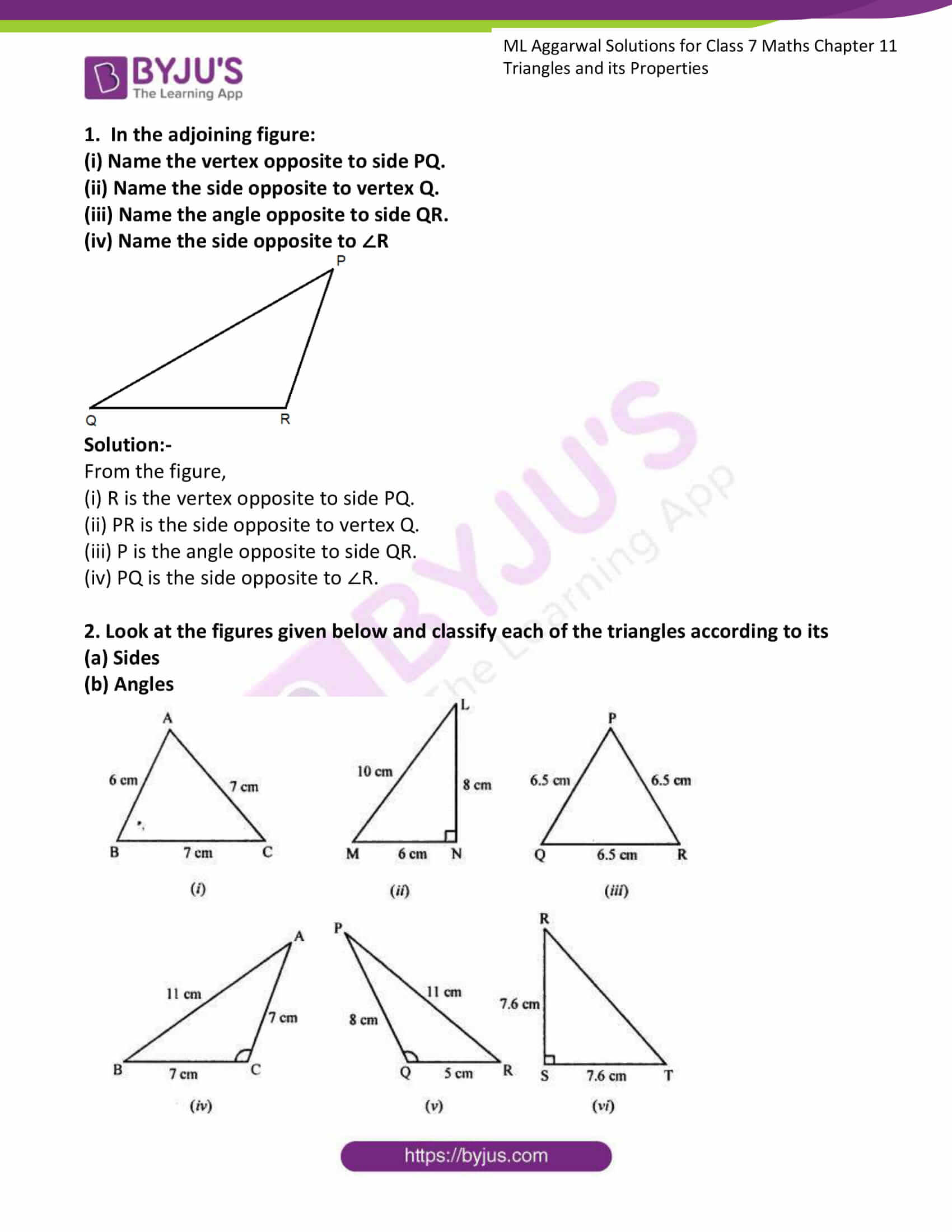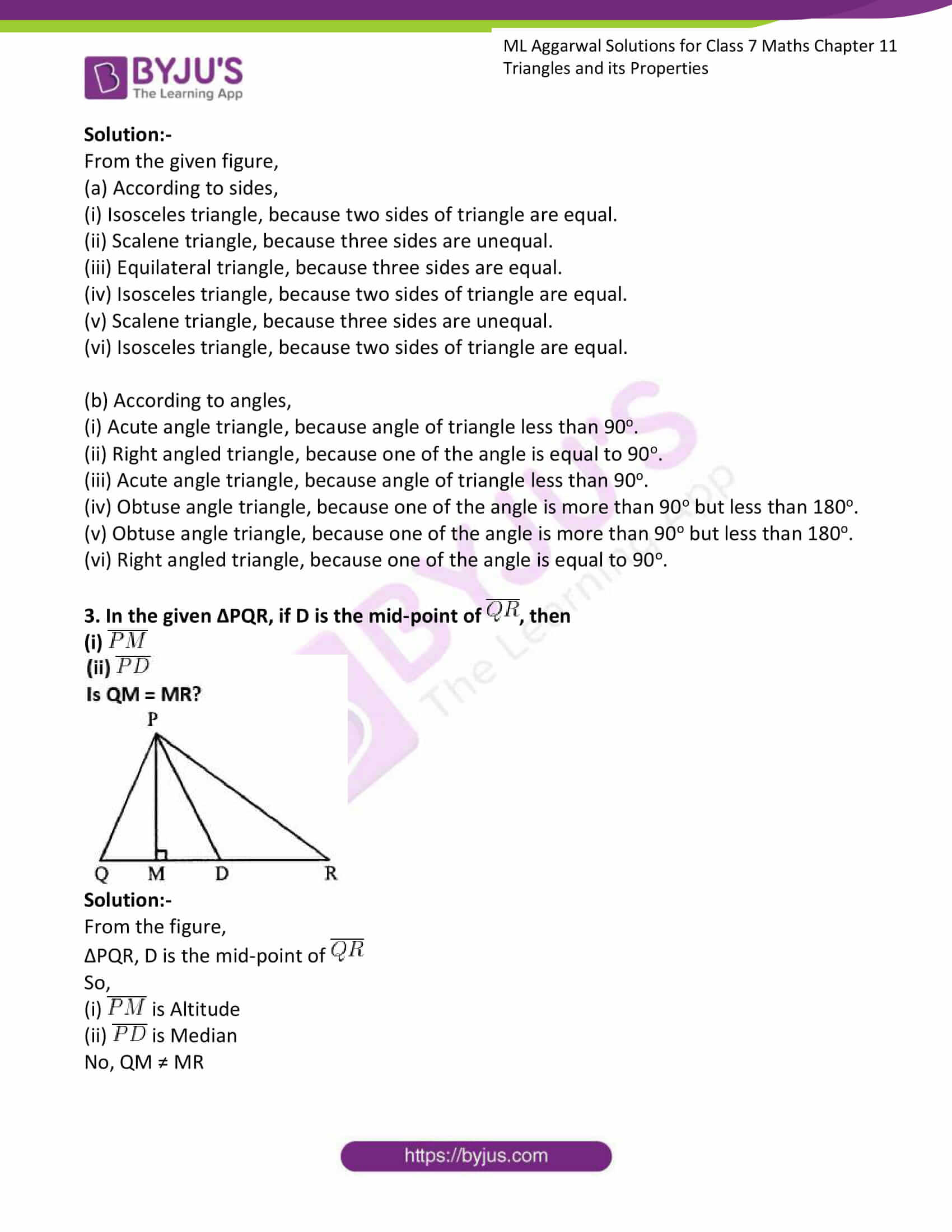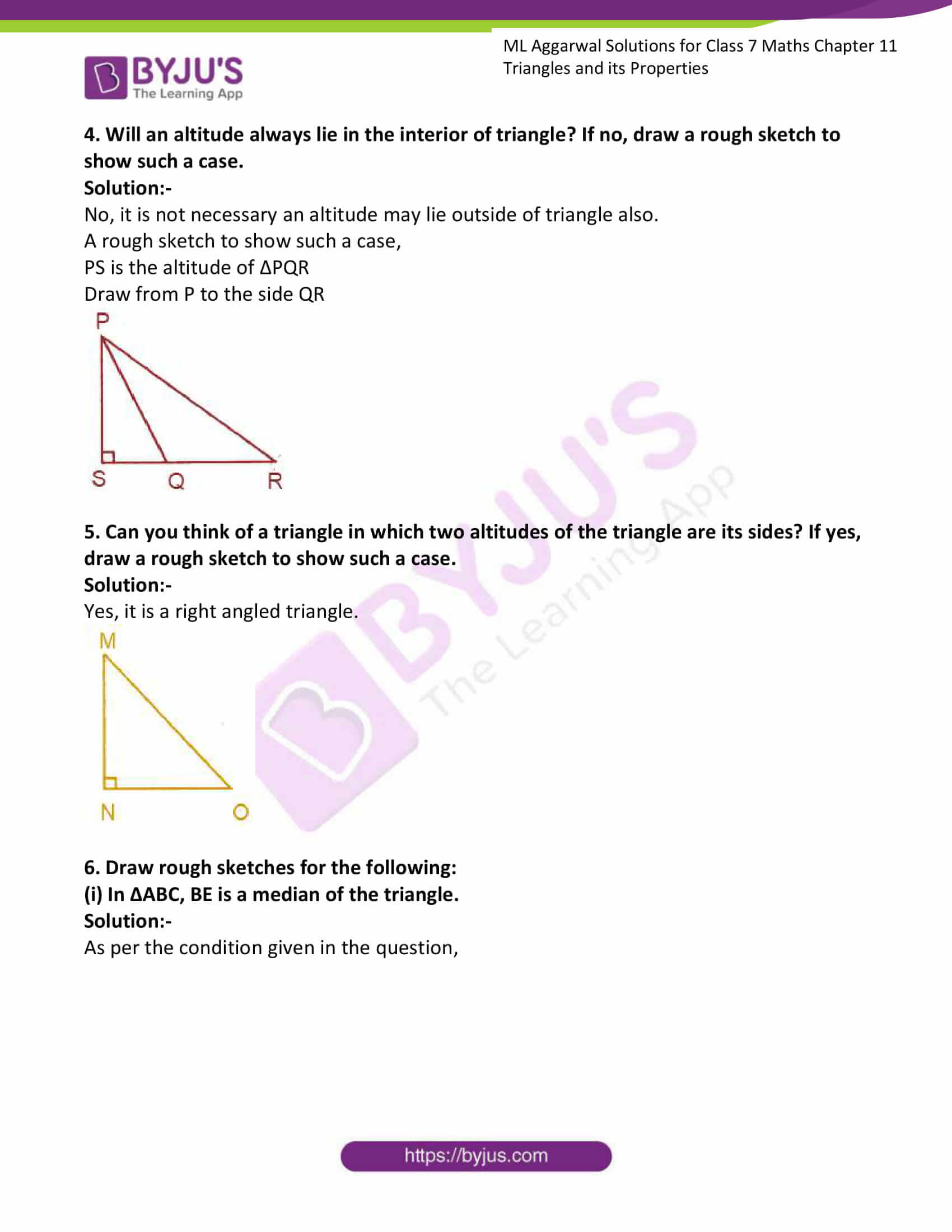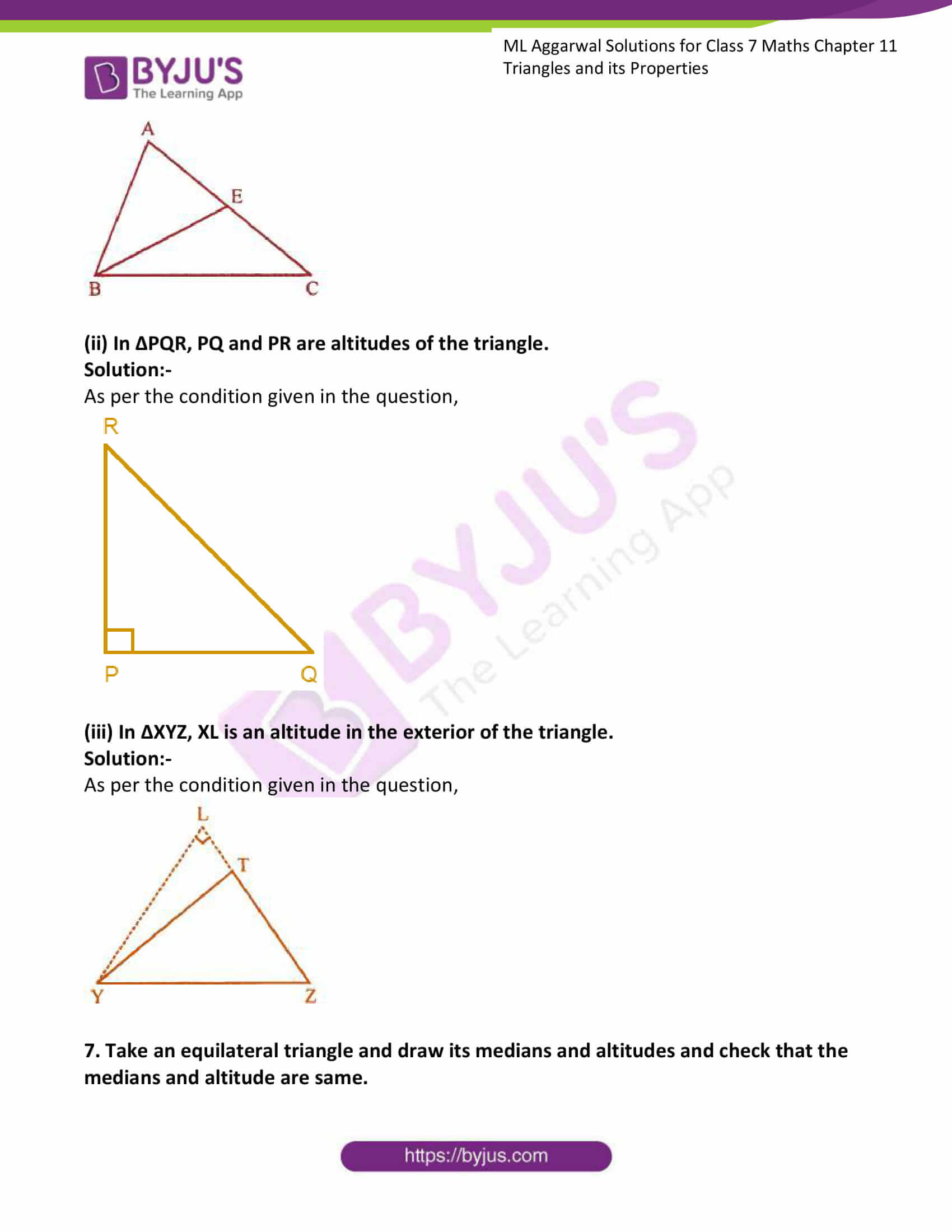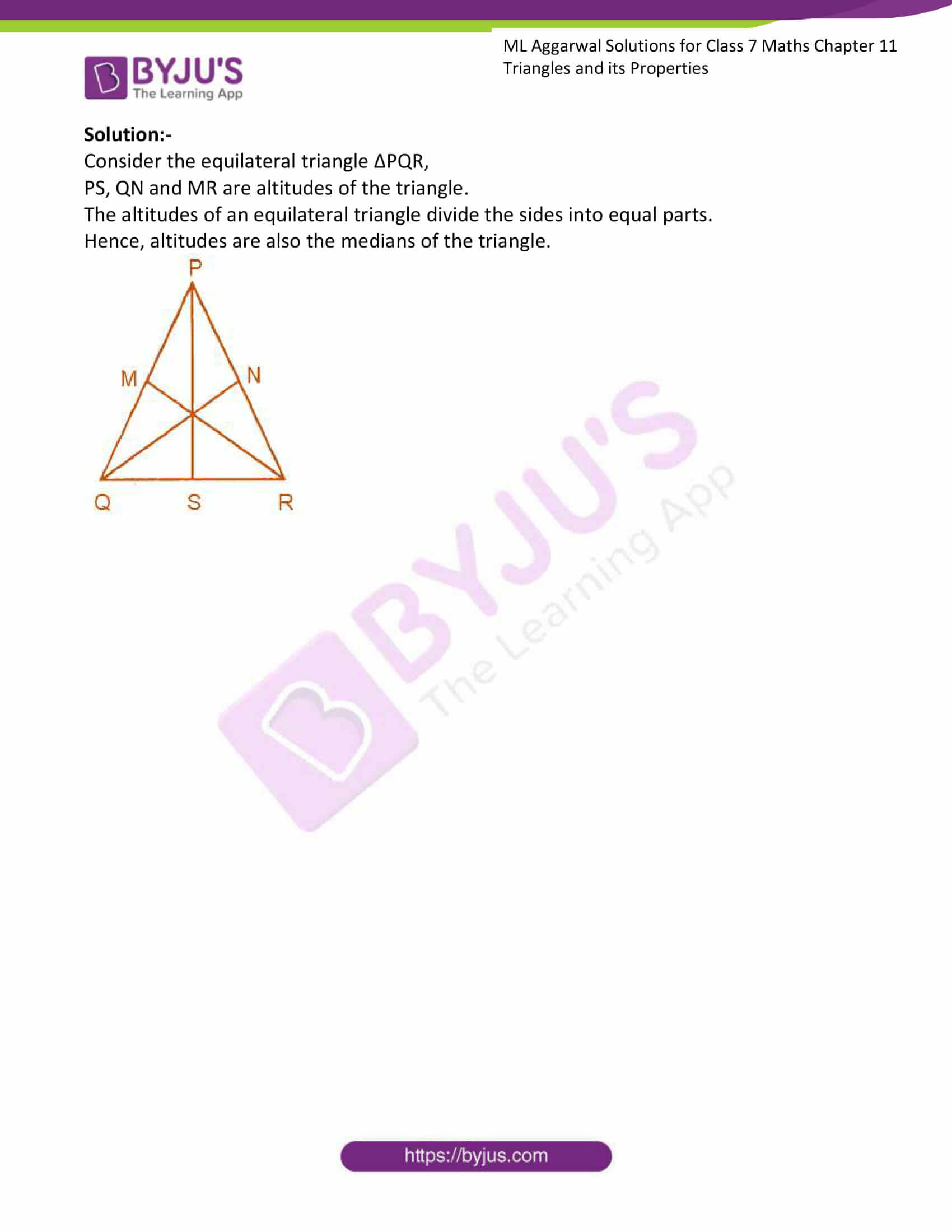### Access answers to ML Aggarwal Solutions for Class 7 Maths Chapter 11 Triangles and its Properties

(i) Name the vertex opposite to side PQ.
(ii) Name the side opposite to vertex Q.
(iii) Name the angle opposite to side QR.
(iv) Name the side opposite to ∠R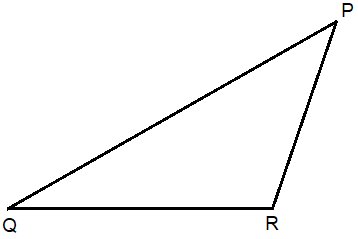Solution:-

From the figure,

(i) R is the vertex opposite to side PQ.

(ii) PR is the side opposite to vertex Q.

(iii) P is the angle opposite to side QR.

(iv) PQ is the side opposite to ∠R.

2. Look at the figures given below and classify each of the triangles according to its

(a) Sides

(b) Angles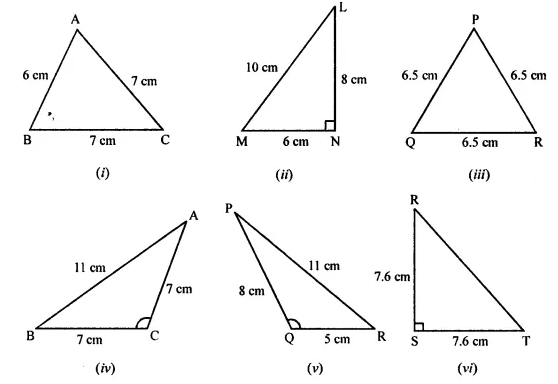Solution:-

From the given figure,

(a) According to sides,

(i) Isosceles triangle, because two sides of triangle are equal.

(ii) Scalene triangle, because three sides are unequal.

(iii) Equilateral triangle, because three sides are equal.

(iv) Isosceles triangle, because two sides of triangle are equal.

(v) Scalene triangle, because three sides are unequal.

(vi) Isosceles triangle, because two sides of triangle are equal.

(b) According to angles,

(i) Acute angle triangle, because angle of triangle less than 90o.

(ii) Right angled triangle, because one of the angle is equal to 90o.

(iii) Acute angle triangle, because angle of triangle less than 90o.

(iv) Obtuse angle triangle, because one of the angle is more than 90o but less than 180o.

(v) Obtuse angle triangle, because one of the angle is more than 90o but less than 180o.

(vi) Right angled triangle, because one of the angle is equal to 90o.

3. In the given ∆PQR, if D is the mid-point of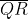, then

(i)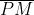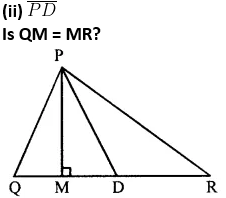Solution:-

From the figure,

∆PQR, D is the mid-point ofSo,

(i)is Altitude

(ii)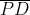is Median

No, QM ≠ MR

4. Will an altitude always lie in the interior of triangle? If no, draw a rough sketch to show such a case.

Solution:-

No, it is not necessary an altitude may lie outside of triangle also.

A rough sketch to show such a case,

PS is the altitude of ∆PQR

Draw from P to the side QR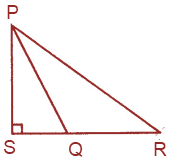5. Can you think of a triangle in which two altitudes of the triangle are its sides? If yes, draw a rough sketch to show such a case.

Solution:-

Yes, it is a right angled triangle.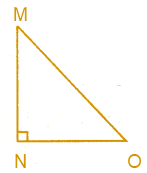6. Draw rough sketches for the following:
(i) In ΔABC, BE is a median of the triangle.

Solution:-

As per the condition given in the question,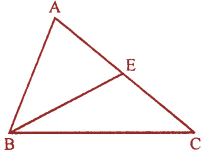(ii) In ΔPQR, PQ and PR are altitudes of the triangle.

Solution:-

As per the condition given in the question,(iii) In ΔXYZ, XL is an altitude in the exterior of the triangle.

Solution:-

As per the condition given in the question,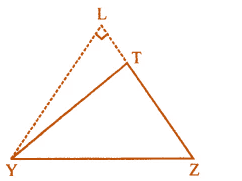7. Take an equilateral triangle and draw its medians and altitudes and check that the medians and altitude are same.

Solution:-

Consider the equilateral triangle ΔPQR,

PS, QN and MR are altitudes of the triangle.

The altitudes of an equilateral triangle divide the sides into equal parts.

Hence, altitudes are also the medians of the triangle.